12 篇文章 2 订阅

1. 引言

1.1 机器学习能够解决的问题

• 我想要回答的问题是什么？已经收集到的数据能够回答这个问题吗？
• 要将我的问题表示成机器学习问题，用哪种方法最好？
• 我收集的数据是否足够表达我想要解决的问题？
• 我提取了数据的哪些特征？这些特征能否实现正确的预测？
• 如何衡量应用是否成功？
• 机器学习解决方案与我的研究或商业产品中的其他部分是如何相互影响的？

1.3 scikit-learn

scikit-learn 是一个开源项目，可以免费使用和分发，任何人都可以轻松获取其源代码来查看其背后的原理

scikit-learn 依赖于另外两个Python 包：NumPy 和SciPy。若想绘图和进行交互式开发，还应该安装matplotlib、IPython 和Jupyter Notebook。

pip install numpy scipy matplotlib ipython scikit-learn pandas

1.4 必要的库和工具

Jupyter notebook、Numpy、Pandas略

1.4.3 Scipy

SciPy 是Python 中用于科学计算的函数集合，具有线性代数高级程序、数学函数优化、信号处理、特殊数学函数和统计分布等多项功能

SciPy 中最重要的是scipy.sparse：它可以给出稀疏矩阵（sparse matrice），稀疏矩阵是scikit-learn 中数据的另一种表示方法

from scipy import sparse
import numpy as np

eye = np.eye(4)
print("Numpy array:\n{}".format(eye))

Numpy array:
[[1. 0. 0. 0.]
[0. 1. 0. 0.]
[0. 0. 1. 0.]
[0. 0. 0. 1.]]

# 将NumPy数组转换为CSR格式的SciPy稀疏矩阵
# 只保存非零元素
sparse_matrix = sparse.csr_matrix(eye)
print("\nScipy sparse CSR matrix:\n{}".format(sparse_matrix))

Scipy sparse CSR matrix:
(0, 0) 1.0
(1, 1) 1.0
(2, 2) 1.0
(3, 3) 1.0

1.4.4 matplotlib

import matplotlib.pyplot as plt
%matplotlib inline

x = np.linspace(-10,10,100)
y = np.sin(x)
plt.plot(x,y,marker='x')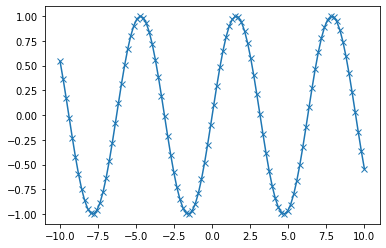1.4.6 mglearn

mglearn是专为本书案例用的库

#安装
pip install mglearn

#导入
import mglearn

1.7 第一个应用：鸢尾花分类

1. 因为我们有已知品种的鸢尾花的测量数据，所以这是一个监督学习问题
2. 要在多个选项中预测其中一个（鸢尾花的品种），这是一个分类（classification）问题的示例
3. 可能的输出（鸢尾花的不同品种）叫作类别（class）。数据集中的每朵鸢尾花都属于三个类别之一，所以这是一个三分类问题
4. 单个数据点（一朵鸢尾花）的预期输出是这朵花的品种。对于一个数据点来说，它的品种叫作标签（label）。

1.7.1 初识数据

#数据集的简要说明
print(iris_dataset.DESCR[:150])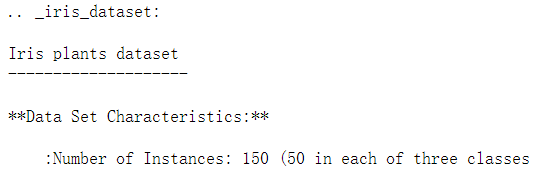#数据集形状、属性、数据
iris_dataset.data.shape
print(iris_dataset.feature_names)
iris_dataset.data[:5]

(150, 4)

[‘sepal length (cm)’, ‘sepal width (cm)’, ‘petal length (cm)’, ‘petal width (cm)’]

array([[5.1, 3.5, 1.4, 0.2],
[4.9, 3. , 1.4, 0.2],
[4.7, 3.2, 1.3, 0.2],
[4.6, 3.1, 1.5, 0.2],
[5. , 3.6, 1.4, 0.2]])

#标签形状、标签名、数据
iris_dataset.target.shape
print(iris_dataset.target_names)
iris_dataset.target

(150,)

[‘setosa’ ‘versicolor’ ‘virginica’]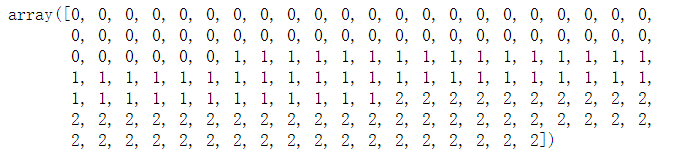data = pd.DataFrame(iris_dataset.data,columns=iris_dataset.feature_names)
data['category'] = iris_dataset.target
data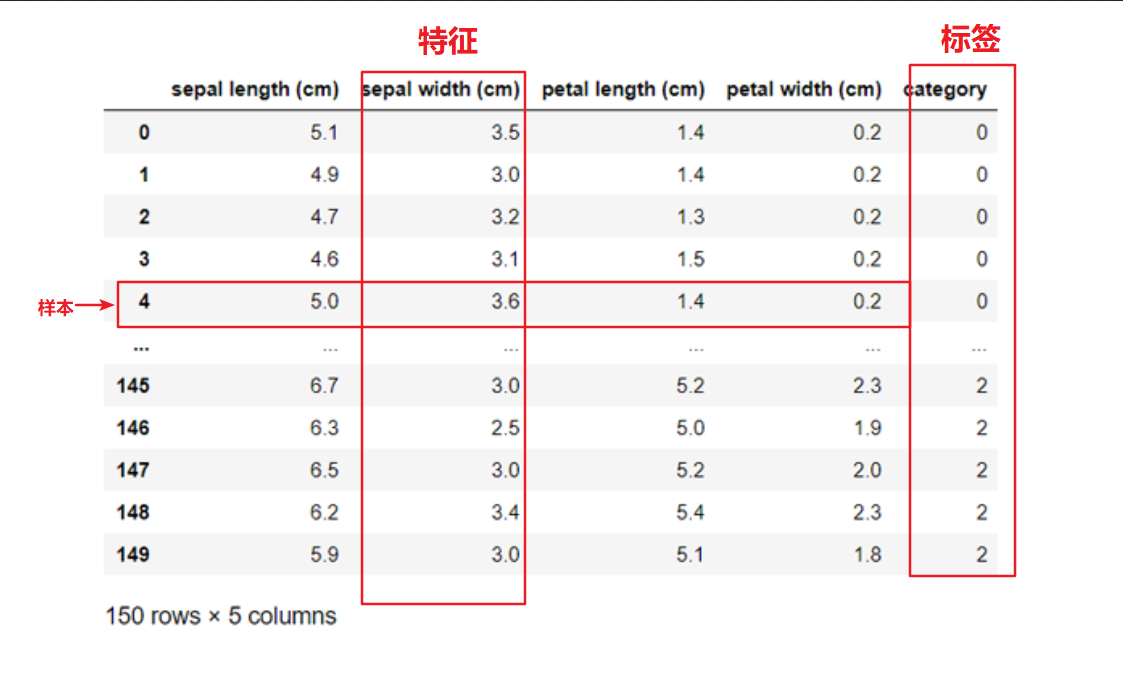1.7.2 衡量模型是否成功：训练数据与测试数据

train_test_split 函数：scikit-learn 中的train_test_split 函数可以打乱数据集并进行拆分。

train_test_split 函数默认是将数据75%分为训练集，25%分为测试集

scikit-learn 中的数据通常用大写的X 表示，而标签用小写的y表示

from sklearn.model_selection import train_test_split
Xtrain,Xtest,ytrain,ytest = train_test_split(iris_dataset.data,iris_dataset.target,random_state=0)

Xtrain.shape,ytrain.shape
Xtest.shape,ytest.shape

((112, 4), (112,))

((38, 4), (38,))

1.7.3　要事第一：观察数据

# 利用X_train中的数据创建DataFrame
# 利用iris_dataset.feature_names中的字符串对数据列进行标记

iris_dataframe = pd.DataFrame(Xtrain,columns=iris_dataset.feature_names)
grr = pd.plotting.scatter_matrix(iris_dataframe,figsize=(15,15),marker='o',hist_kwds={'bins':20},alpha=0.8,cmap=mglearn.cm3)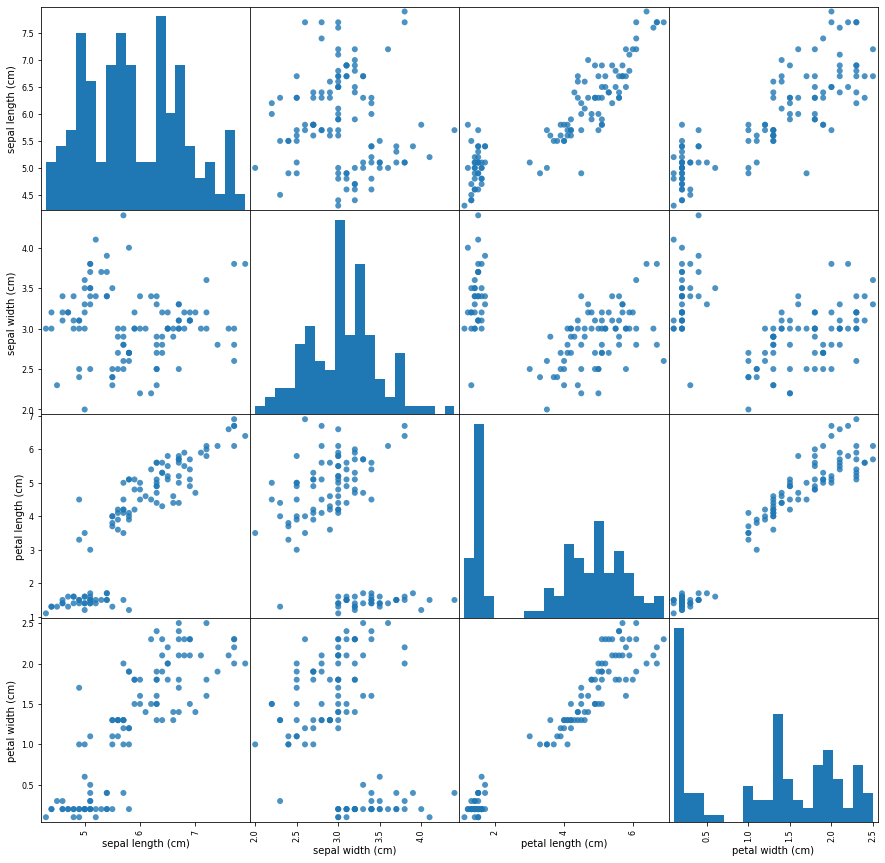1.7.4　构建第一个模型：k近邻算法

scikit-learn 中所有的机器学习模型都在各自的类中实现，这些类被称为Estimator类

k 近邻分类算法是在neighbors 模块的KNeighborsClassifier 类中实现的

from sklearn.neighbors import KNeighborsClassifier
knn = KNeighborsClassifier(n_neighbors=1)

knn.fit(Xtrain,ytrain)

scikit-learn 中的大多数模型都有很多参数，但多用于速度优化或非常特殊的用途,你无需关注这个字符串表示中的其他参数

1.7.5 做出预测

X_new = np.array([[5,2.9,1,0.2]])

prediction = knn.predict(X_new)
print("Predictinon:{}".format(prediction))
print("Predicted target name:{}".format(iris_dataset.target_names[prediction]))

Predictinon:
Predicted target name:[‘setosa’]

1.7.6 评估模型

print("测试精度:{:.2f}".format(knn.score(Xtest,ytest)))

2. 监督学习

2.1 分类与回归

2.1.1 分类问题

classification）：在两个以上的类别之间进行区分

2.3 监督学习算法

2.3.2 k近邻

1. k近邻分类

mglearn.plots.plot_knn_classification(n_neighbors=3)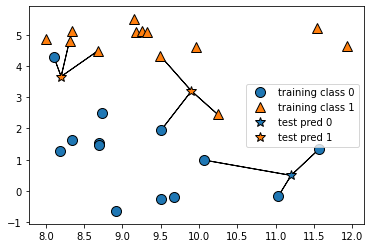#数据集分割
from sklearn.model_selection import train_test_split
X_train,X_test,y_train,y_test = train_test_split(X,y,random_state=0)

#knn实例化
from sklearn.neighbors import KNeighborsClassifier
clf = KNeighborsClassifier(n_neighbors=3)

#训练模型
clf.fit(X_train,y_train)

#模型预测
clf.predict(X_test)

#模型得分
clf.score(X_test,y_test)

KNeighborsClassifier(n_neighbors=3)

array([1, 0, 1, 0, 1, 0, 0])

0.8571428571428571

2. 分析KNeighborsClassifier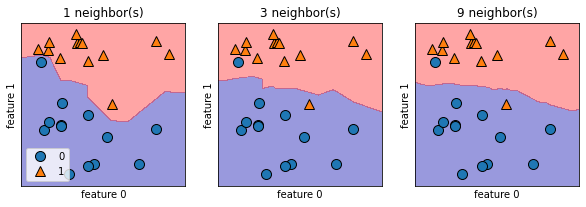from matplotlib import pyplot as plt
%matplotlib inline
import pandas as pd
from sklearn.model_selection import train_test_split
from sklearn.neighbors import KNeighborsClassifier

#导入数据
X = cancer.data
y = cancer.target

#拆分数据集
X_train,X_test,y_train,y_test = train_test_split(
X,y,random_state=0)

neighbors_setting = range(1,11)
train_accuracy = []
test_accuracy = []

#设置不同邻居时，得到得分
for n_neighbors in neighbors_setting:
clf = KNeighborsClassifier(n_neighbors=n_neighbors)
clf.fit(X_train,y_train)
train_accuracy.append(clf.score(X_train,y_train))
test_accuracy.append(clf.score(X_test,y_test))

plt.rcParams['font.sans-serif'] = 'SimHei'
#得分进行画图
plt.plot(neighbors_setting,train_accuracy,label="训练精度")
plt.plot(neighbors_setting,test_accuracy,label="测试精度")
plt.xlabel("近邻n值")
plt.ylabel("精度")
plt.legend()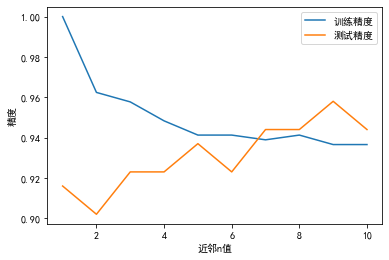3. k近邻回归

from sklearn.neighbors import KNeighborsRegressor

X,y = mglearn.datasets.make_wave(n_samples=40)
# 将wave数据集分为训练集和测试集
X_train,X_test,y_train,y_test = train_test_split(X,y,random_state=0)

# 模型实例化，并将邻居个数设为3
reg = KNeighborsRegressor(n_neighbors=3)
# 利用训练数据和训练目标值来拟合模型
reg.fit(X_train,y_train)

#模型预测
reg.predict(X_test)
#模型得分
reg.score(X_test,y_test)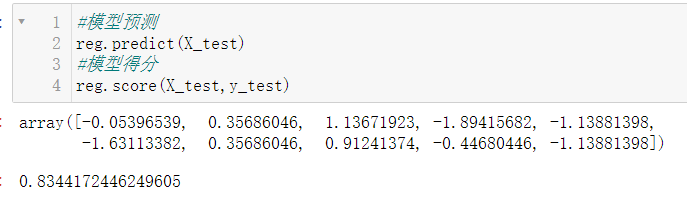4. 分析KNeighborsRegressor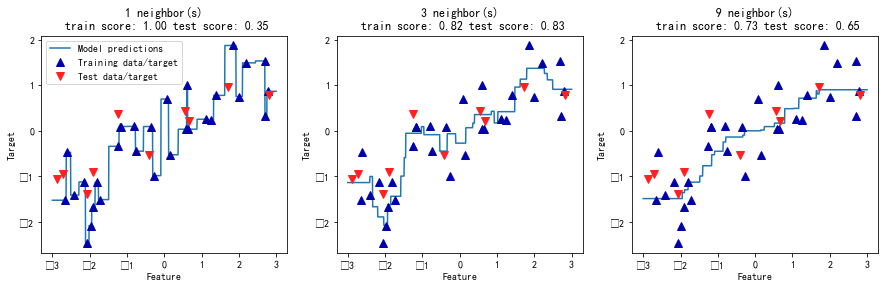优点、缺点和参数

KNeighbors 分类器使用较小的邻居个数（比如3 个或5个）往往可以得到比较好的结果，但应

1. 不能处理具有很多特征的数据集
2. 预测速度可能会比较慢

2.3.3 线性模型

1. 用于回归的线性模型

y ^ = w [ 0 ] ∗ x [ 0 ] + w [ 1 ] ∗ x [ 1 ] + . . . + w [ p ] ∗ x [ p ] + b \hat{y} = w*x + w*x + ... + w[p]*x[p] + b

x 到x[p] 表示单个数据点的特征（本例中特征个数为p+1），w 和b 是学习模型的参数，ŷ 是模型的预测结果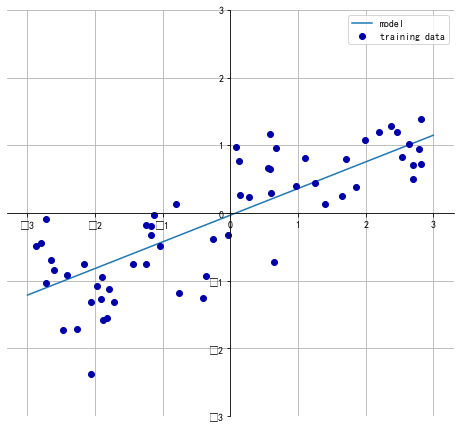2. 线性回归（又名普通最小二乘法，OLS）

from sklearn.linear_model import LinearRegression

X,y = mglearn.datasets.make_wave(n_samples=60)
X_train, X_test, y_train, y_test = train_test_split(X, y, random_state=42)

X,y = mglearn.datasets.make_wave(n_samples=60)
X_train, X_test, y_train, y_test = train_test_split(X, y, random_state=42)

lr = LinearRegression().fit(X_train,y_train)

print("斜率w: {}".format(lr.coef_))
print("截距b: {}".format(lr.intercept_))

intercept_ 属性是一个浮点数，而coef_ 属性是一个NumPy 数组，每个元素对应一个输入特征

X_train, X_test, y_train, y_test = train_test_split(X, y, random_state=0)
lr = LinearRegression().fit(X_train, y_train)

print("Training set score: {:.2f}".format(lr.score(X_train, y_train)))
print("Test set score: {:.2f}".format(lr.score(X_test, y_test)))

Training set score: 0.95
Test set score: 0.61

3. 岭回归(L2)

from sklearn.linear_model import Ridge

ridge = Ridge().fit(X_train,y_train)
print("Training set score: {:.2f}".format(ridge.score(X_train, y_train)))
print("Test set score: {:.2f}".format(ridge.score(X_test, y_test)))

Training set score: 0.89
Test set score: 0.75

Ridge 在训练集上的分数要低于LinearRegression，但在测试集上的分数更高

Ridge函数通过调整alpha值（默认为1），来找到训练集性能与泛化能力之间的平衡——alpha越大，系数越趋向于0，泛化性能越强，训练集降低

ridge = Ridge(alpha=10).fit(X_train,y_train)
print("Training set score: {:.2f}".format(ridge.score(X_train, y_train)))
print("Test set score: {:.2f}".format(ridge.score(X_test, y_test)))

Training set score: 0.79
Test set score: 0.64

ridge = Ridge(alpha=0.1).fit(X_train,y_train)
print("Training set score: {:.2f}".format(ridge.score(X_train, y_train)))
print("Test set score: {:.2f}".format(ridge.score(X_test, y_test)))

Training set score: 0.93
Test set score: 0.77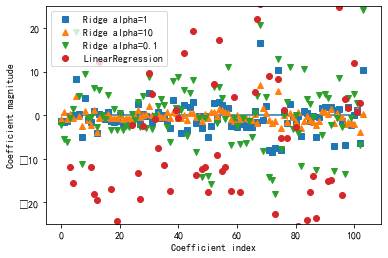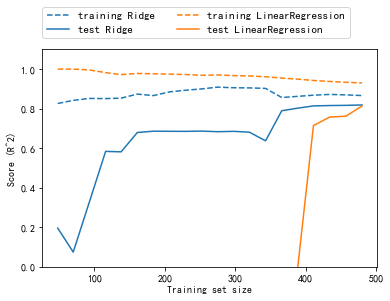4. lasso(L1)

lasso也是约束系数使其接近于0，但用到的方法不同，叫作L1正则化

from sklearn.linear_model import Lasso
lasso = Lasso().fit(X_train, y_train)

print("Training set score: {:.2f}".format(lasso.score(X_train, y_train)))
print("Test set score: {:.2f}".format(lasso.score(X_test, y_test)))
print("Number of features used: {}".format(np.sum(lasso.coef_ != 0)))

Training set score: 0.29
Test set score: 0.21
Number of features used: 4

Lasso在训练集与测试集上存在欠拟合，我们发现模型只用到了105 个特征中的4个

Lasso 通过alpha控制系数趋向于0的强度，减小alpha，同时增加max_iter 的值（运行迭代的最大次数），可以降低欠拟合。

# 我们增大max_iter的值，否则模型会警告我们，说应该增大max_iter
lasso001 = Lasso(alpha=0.01,max_iter=100000).fit(X_train,y_train)
print("Training set score: {:.2f}".format(lasso001.score(X_train, y_train)))
print("Test set score: {:.2f}".format(lasso001.score(X_test, y_test)))
print("Number of features used: {}".format(np.sum(lasso001.coef_ != 0)))

Training set score: 0.90
Test set score: 0.77
Number of features used: 33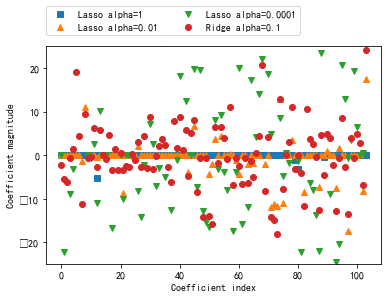5. 用于二分类的线性模型

y ^ = w [ 0 ] ∗ x [ 0 ] + w [ 1 ] ∗ x [ 1 ] + . . . + w [ p ] ∗ x [ p ] + b > 0 \hat{y} = w*x + w*x + ... + w[p]*x[p] + b> 0

• 用于回归的线性模型，输出ŷ 是特征的线性函数，是直线、平面或超平面（对于更高维的数据集）。
• 对于用于分类的线性模型，（二元）线性分类器是利用直线、平面或超平面，来分开两个类别的分类器

• 系数和截距的特定组合对训练数据拟合好坏的度量方法；
• 是否使用正则化，以及使用哪种正则化方法。

from sklearn.linear_model import LogisticRegression
from sklearn.svm import LinearSVC

X, y = mglearn.datasets.make_forge()

fig, axes = plt.subplots(1, 2, figsize=(10, 3))

for model, ax in zip([LinearSVC(), LogisticRegression()], axes):
clf = model.fit(X, y)
mglearn.plots.plot_2d_separator(clf, X, fill=False, eps=0.5,
ax=ax, alpha=.7)
mglearn.discrete_scatter(X[:, 0], X[:, 1], y, ax=ax)
ax.set_title("{}".format(clf.__class__.__name__))
ax.set_xlabel("Feature 0")
ax.set_ylabel("Feature 1")
axes.legend()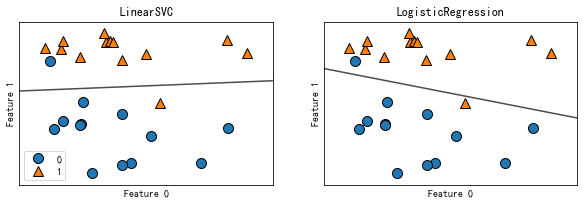1. 都默认使用L2 正则化
2. 决定正则化强度的权衡参数叫作C。C 值越大，对应的正则化越弱，将尽可能将训练集拟合到最好；C 值较小，那么模型更强调使系数向量（w）接近于0
3. 较小的C 值可以让算法尽量适应“大多数”数据点，而较大的C 值更强调每个数据点都分类正确的重要性。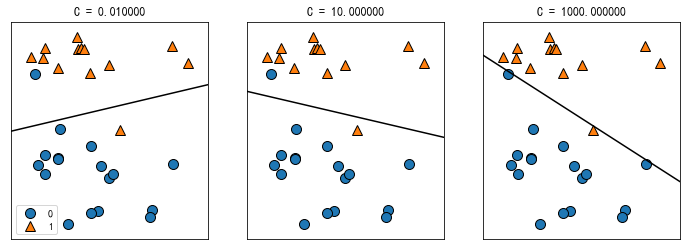X_train, X_test, y_train, y_test = train_test_split(
cancer.data, cancer.target, stratify=cancer.target, random_state=42)
LR = LogisticRegression().fit(X_train,y_train)
print("Training set score: {:.3f}".format(LR.score(X_train, y_train)))
print("Test set score: {:.3f}".format(LR.score(X_test, y_test)))

Training set score: 0.946
Test set score: 0.958

LR100 = LogisticRegression(C=100).fit(X_train, y_train)
print("Training set score: {:.3f}".format(LR100.score(X_train, y_train)))
print("Test set score: {:.3f}".format(LR100.score(X_test, y_test)))

Training set score: 0.948
Test set score: 0.965

LR001 = LogisticRegression(C=0.01).fit(X_train, y_train)
print("Training set score: {:.3f}".format(LR001.score(X_train, y_train)))
print("Test set score: {:.3f}".format(LR001.score(X_test, y_test)))

Training set score: 0.934
Test set score: 0.930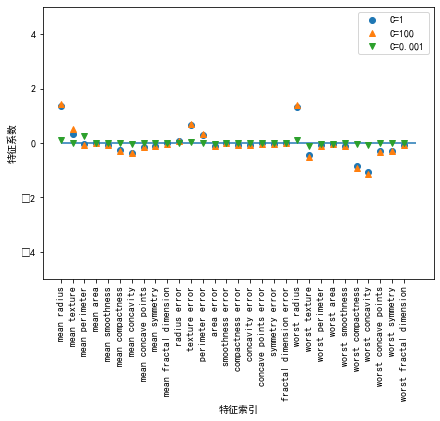6. 用于多分类的线性模型

01-2003-16242
01-14
12-173914
12-152167
03-08674
04-086585
04-026863
09-049万+
03-24930
08-02131
04-283671
03-072904
08-025605
12-124403
03-141万+
02-06660¥2 ¥4 ¥6 ¥10 ¥20余额支付 (余额：-- )扫码支付获取中扫码支付点击重新获取扫码支付1.余额是钱包充值的虚拟货币，按照1:1的比例进行支付金额的抵扣。
2.余额无法直接购买下载，可以购买VIP、C币套餐、付费专栏及课程。余额充值